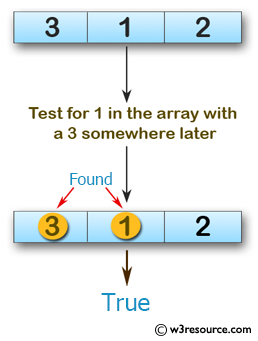﻿ Swift Array Programming Exercise: Test if there is a 1 in the array with a 3 somewhere later in a given array of integers - w3resource# Swift Array Programming Exercises: Test if there is a 1 in the array with a 3 somewhere later in a given array of integers

## Swift Array Programming: Exercise-33 with Solution

Write a Swift program to test if there is a 1 in the array with a 3 somewhere later in a given array of integers.

Pictorial Presentation:Sample Solution:

Swift Code:

``````func num13(array_nums: [Int]) -> Bool {
for num in array_nums {
if num == 1 {
for num in array_nums {
if num == 3
{
return true
}
}
}
}
return false
}

print(num13(array_nums: [0, 3, 2]))
print(num13(array_nums: [3, 1, 2]))
print(num13(array_nums: [3, 1, 7, 5, 2]))
```
```

Sample Output:

```false
true
true
```

Swift Programming Code Editor:

Improve this sample solution and post your code through Disqus

What is the difficulty level of this exercise?

﻿